# Models of user engagement

Jul. 18, 2012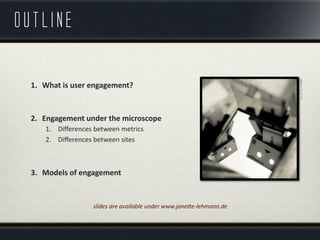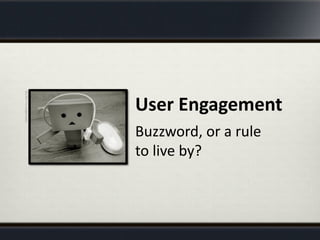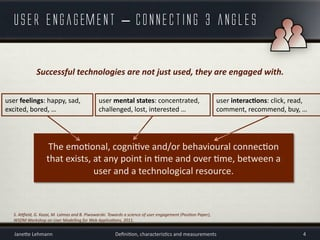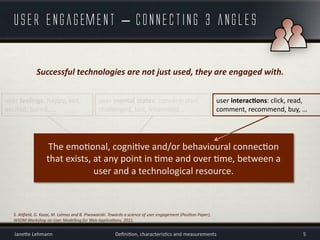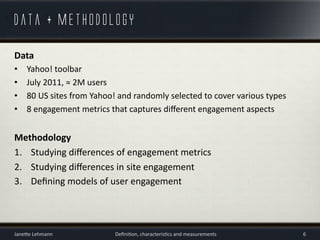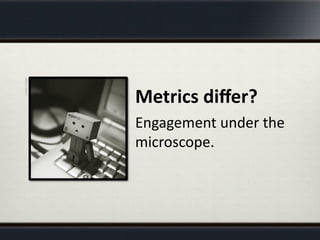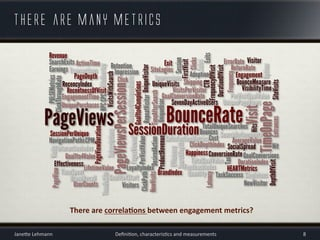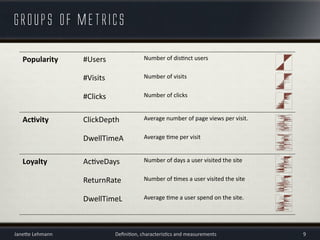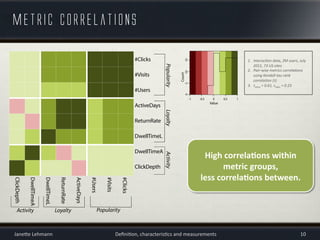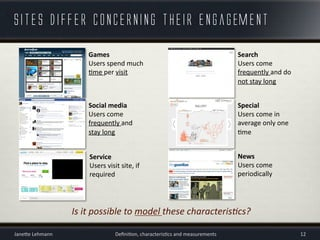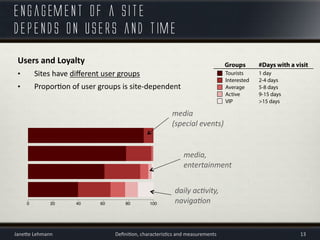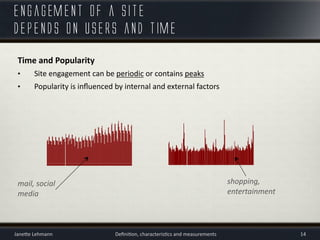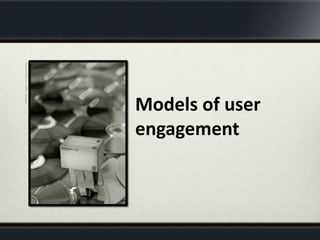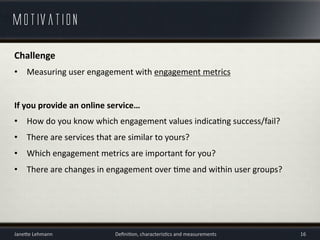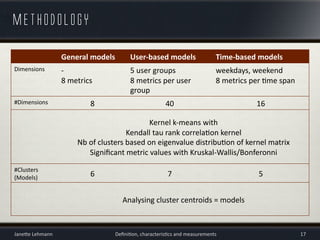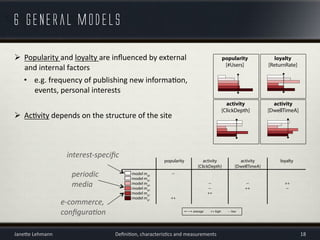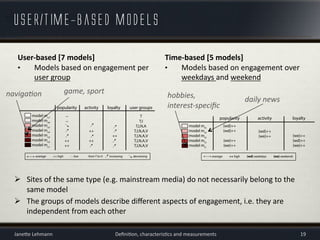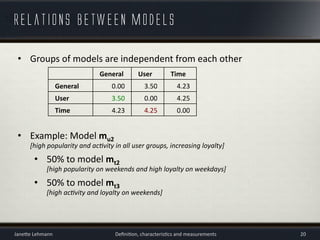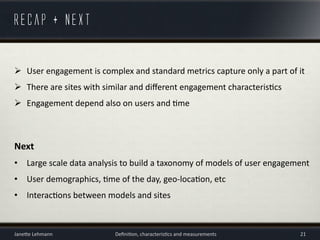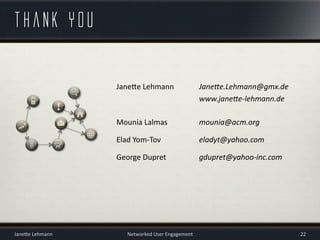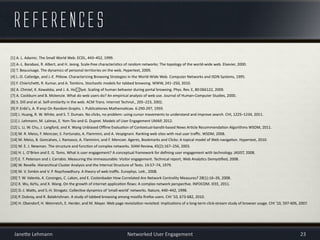1 of 23

### Models of user engagement

1. !"#\$%\$&'\$()"##& !"#\$%&&'(%)'*%"#+,-./# 0%1",'#2'&3'45#6&'7#8%39:%;5## <=%)>=#?1@)=-#
2. 1\$23-*+4(+,5+6789:;+ !"  #\$%&'()'*)+,'+-.%.+/+-&0' 1"  2-.%.+/+-&'*-3+,'&\$+'/(4,5)456+' !"  #\$%&'&()&*+,&-.&&(+/&-'\$)*+ 0"  #\$%&'&()&*+,&-.&&(+*\$-&*+ 7"  853+9)'5:'+-.%.+/+-&' 4&,7=4#')=#';',&'(&=#1"7=)#+++AB'"=C=9&=.3'""A7=#
3. !<0=>?@9!+,5+/&A2A(/AB&*+ ;)+,'2-.%.+/+-&' CDEE.4'FG+4'+A+'DH&++ -4+H\$I&+,5J+
4. *+,,\$--.+/&0\$,(#1/123\$-&"4\$&#10&5+-0&+-\$67&0(\$8&"4\$&\$#2"2\$6&930(:& D*&'+:++9(-.)M+3ANN5G+*AFG+ D*&'+/+-&%9')&%&+)M+)4()&(-'A-&FG+ D*&'+(-&+,%4<5-)M+)H\$)BG+'&AFG+ &O)\$-&FG+,4'&FG+P+ )3AHH&(2&FG+H4*-G+\$(-&'&*-&F+P+ )4//&(-G+'&)4//&(FG+,D5G+P+ Q3&+&/4R4(AHG+)42(\$RI&+A(FS4'+,&3AI\$4D'AH+)4((&)R4(++ -3A-+&O\$*-*G+A-+A(5+N4\$(-+\$(+R/&+A(F+4I&'+R/&G+,&-.&&(+A++ D*&'+A(F+A+-&)3(4H42\$)AH+'&*4D')&"+ DA#EF,=&75#<A#G'H',5#0A#2'&3'4#'"7#IA#J,+%+')4K,A#:%+')74#'#4\$,="\$=#%L#14=)#=">'>=3="-#MJ%4,*%"#J'@=)N5## OD?0#O%)K4.%@#%"#P4=)#0%7=&&,">#L%)#O=(#E@@&,\$'*%"45#QRSSA# 6A(&L&+1&3/A((+ #&T(\$R4(G+)3A'A)-&'\$*R)*+A(F+/&A*D'&/&(-*+ K+
5. *+,,\$--.+/&0\$,(#1/123\$-&"4\$&#10&5+-0&+-\$67&0(\$8&"4\$&\$#2"2\$6&930(:& D*&'+:++9(-.)M+3ANN5G+*AFG+ D*&'+/+-&%9')&%&+)M+)4()&(-'A-&FG+ D*&'+(-&+,%4<5-)M+)H\$)BG+'&AFG+ &O)\$-&FG+,4'&FG+P+ )3AHH&(2&FG+H4*-G+\$(-&'&*-&F+P+ )4//&(-G+'&)4//&(FG+,D5G+P+ Q3&+&/4R4(AHG+)42(\$RI&+A(FS4'+,&3AI\$4D'AH+)4((&)R4(++ -3A-+&O\$*-*G+A-+A(5+N4\$(-+\$(+R/&+A(F+4I&'+R/&G+,&-.&&(+A++ D*&'+A(F+A+-&)3(4H42\$)AH+'&*4D')&"+ DA#EF,=&75#<A#G'H',5#0A#2'&3'4#'"7#IA#J,+%+')4K,A#:%+')74#'#4\$,="\$=#%L#14=)#=">'>=3="-#MJ%4,*%"#J'@=)N5## OD?0#O%)K4.%@#%"#P4=)#0%7=&&,">#L%)#O=(#E@@&,\$'*%"45#QRSSA# 6A(&L&+1&3/A((+ #&T(\$R4(G+)3A'A)-&'\$*R)*+A(F+/&A*D'&/&(-*+ @+
6. =%&%' •  UA344;+-44H,A'+ •  6DH5+0V!!G+W+0X+D*&'*+ •  <V+YZ+*\$-&*+['4/+UA344;+A(F+'A(F4/H5+*&H&)-&F+-4+)4I&'+IA'\$4D*+-5N&*+ •  <+&(2A2&/&(-+/&-'\$)*+-3A-+)AN-D'&*+F\$%&'&(-+&(2A2&/&(-+A*N&)-*+ 8+&\$53595.>' !"  Z-DF5\$(2+F\$%&'&()&*+4[+&(2A2&/&(-+/&-'\$)*+ 0"  Z-DF5\$(2+F\$%&'&()&*+\$(+*\$-&+&(2A2&/&(-+ >"  #&T(\$(2+/4F&H*+4[+D*&'+&(2A2&/&(-+ 6A(&L&+1&3/A((+ #&T(\$R4(G+)3A'A)-&'\$*R)*+A(F+/&A*D'&/&(-*+ ?+
7. H&+,5++]+&/,'+^++ 8+&,(4)'3(?+,0' _(2A2&/&(-+D(F&'+-3&+ /\$)'4*)4N&"+
8. '@\$+,+'%,+'45,,+9%<5-)'A+&B++-'+-.%.+/+-&'/+&,(4)0' 6A(&L&+1&3/A((+ #&T(\$R4(G+)3A'A)-&'\$*R)*+A(F+/&A*D'&/&(-*+ <+
9. C56*9%,(&>' aY*&'*+ bD/,&'+4[+F\$*R()-+D*&'*+ ac\$*\$-*+ bD/,&'+4[+I\$*\$-*+ a7H\$)B*+ bD/,&'+4[+)H\$)B*+ D4<E(&>' 7H\$)B#&N-3+ :I&'A2&+(D/,&'+4[+NA2&+I\$&.*+N&'+I\$*\$-"+ #.&HHQ\$/&:+ :I&'A2&+R/&+N&'+I\$*\$-+ F5>%9&>' :)RI&#A5*+ bD/,&'+4[+FA5*+A+D*&'+I\$*\$-&F+-3&+*\$-&+ d&-D'(dA-&+ bD/,&'+4[+R/&*+A+D*&'+I\$*\$-&F+-3&+*\$-&+ #.&HHQ\$/&1+ :I&'A2&+R/&+A+D*&'+*N&(F+4(+-3&+*\$-&"+ 6A(&L&+1&3/A((+ #&T(\$R4(G+)3A'A)-&'\$*R)*+A(F+/&A*D'&/&(-*+ `+
10. #Clicks SA  !"-=)'\$*%"#7'-'5#Q0#14=)45#T1&U# 15 QRSS5#VW#PD#4,-=4# Popularity QA  J',)9+,4=#3=-),\$4#\$%))=&'*%"4# 10 #Visits Count 14,">#G="7'&&#-'1#)'"K# \$%))=&'*%"#MXN# 5 WA  X,"-)'#Y#RAZS5#X,"-=)#Y#RAQW# #Users 0 -1 -0.5 0 0.5 1 Value ActiveDays Loyalty ReturnRate DwellTimeL DwellTimeA G(.\$'45,,+9%<5-)'B(&\$(-'' ClickDepth Activity /+&,(4'.,5*6)H'' 9+))'45,,+9%<5-)'A+&B++-"' ClickDepth DwellTimeA DwellTimeL ReturnRate ActiveDays #Users #Visits #Clicks Activity Loyalty Popularity 6A(&L&+1&3/A((+ #&T(\$R4(G+)3A'A)-&'\$*R)*+A(F+/&A*D'&/&(-*+ !V+
11. _I&'5-3\$(2+\$*+*BA-&A,H&""+,5+:efgK+ I(&+)'3(?+,0' _(2A2&/&(-+D(F&'+-3&+ /\$)'4*)4N&"+
12. J%/+)' I+%,4\$' Y*&'*+*N&(F+/D)3+ Y*&'*+)4/&+ R/&+N&'+I\$*\$-+ ['&hD&(-H5+A(F+F4+ (4-+*-A5+H4(2+ I54(%9'/+3(%' I6+4(%9' Y*&'*+)4/&+ Y*&'*+)4/&+\$(+ ['&hD&(-H5+A(F+ AI&'A2&+4(H5+4(&+ *-A5+H4(2+ R/&+ I+,E(4+' K+B)' Y*&'*+I\$*\$-+*\$-&G+\$[+ Y*&'*+)4/&+ '&hD\$'&F+ N&'\$4F\$)AHH5+ !4#,-#@%44,(&=#-%#3%7=&#-.=4=#\$.')'\$-=),4*\$4[& 6A(&L&+1&3/A((+ #&T(\$R4(G+)3A'A)-&'\$*R)*+A(F+/&A*D'&/&(-*+ !0+
13. ;)+,)'%-3'F5>%9&>' Groups #Days with a visit •  Z\$-&*+3AI&+F\$%&'&(-+D*&'+2'4DN*+ Tourists 1 day Interested 2-4 days •  i'4N4'R4(+4[+D*&'+2'4DN*+\$*+*\$-&=F&N&(F&(-+ Average 5-8 days Active 9-15 days VIP >15 days 3=7,'# M4@=\$,'&#=;="-4N# 3=7,'5# ="-=)-',"3="-# 7',&U#'\$*;,-U5# 0 20 40 60 80 100 "';,>'*%"# 6A(&L&+1&3/A((+ #&T(\$R4(G+)3A'A)-&'\$*R)*+A(F+/&A*D'&/&(-*+ !>+
14. @(/+'%-3'C56*9%,(&>' •  Z\$-&+&(2A2&/&(-+)A(+,&+N&'\$4F\$)+4'+)4(-A\$(*+N&AB*+ •  i4NDHA'\$-5+\$*+\$(jD&()&F+,5+\$(-&'(AH+A(F+&O-&'(AH+[A)-4'*+ 3',&5#4%\$,'&# 4.%@@,">5# 3=7,'# ="-=)-',"3="-# 6A(&L&+1&3/A((+ #&T(\$R4(G+)3A'A)-&'\$*R)*+A(F+/&A*D'&/&(-*+ !K+
15. 853+9)'5:'*)+,' +-.%.+/+-&' #A(,4A'F+24&*+[4'+A+.AHB"""+,5+2.AA'++
16. L\$%99+-.+' •  X&A*D'\$(2+D*&'+&(2A2&/&(-+.\$-3+&(2A2&/&(-+/&-'\$)*+ M:'>5*'6,5E(3+'%-'5-9(-+')+,E(4+N' •  k4.+F4+54D+B(4.+.3\$)3+&(2A2&/&(-+IAHD&*+\$(F\$)AR(2+*D))&**S[A\$HJ+ •  Q3&'&+A'&+*&'I\$)&*+-3A-+A'&+*\$/\$HA'+-4+54D'*J+ •  l3\$)3+&(2A2&/&(-+/&-'\$)*+A'&+\$/N4'-A(-+[4'+54DJ+ •  Q3&'&+A'&+)3A(2&*+\$(+&(2A2&/&(-+4I&'+R/&+A(F+.\$-3\$(+D*&'+2'4DN*J+ 6A(&L&+1&3/A((+ #&T(\$R4(G+)3A'A)-&'\$*R)*+A(F+/&A*D'&/&(-*+ !?+
17. J+-+,%9'/53+9)' ;)+,OA%)+3'/53+9)' @(/+OA%)+3'/53+9)' #\$/&(*\$4(*+ =+ @+D*&'+2'4DN*+ .&&BFA5*G+.&&B&(F+ <+/&-'\$)*+ <+/&-'\$)*+N&'+D*&'+ <+/&-'\$)*+N&'+R/&+*NA(+ 2'4DN+ a#\$/&(*\$4(*+ <+ KV+ !?+ e&'(&H+B=/&A(*+.\$-3++ e&(FAHH+-AD+'A(B+)4''&HAR4(+B&'(&H+ b,+4[+)HD*-&'*+,A*&F+4(+&\$2&(IAHD&+F\$*-'\$,DR4(+4[+B&'(&H+/A-'\$O+ Z\$2(\$T)A(-+/&-'\$)+IAHD&*+.\$-3+e'D*BAH=lAHH\$*SC4([&'4((\$+ a7HD*-&'*+ mX4F&H*n+ ?+ g+ @+ :(AH5*\$(2+)HD*-&'+)&(-'4\$F*+o+/4F&H*+ 6A(&L&+1&3/A((+ #&T(\$R4(G+)3A'A)-&'\$*R)*+A(F+/&A*D'&/&(-*+ !g+
18. !  i4NDHA'\$-5+A(F+H45AH-5+A'&+\$(jD&()&F+,5+&O-&'(AH+ popularity loyalty [#Users] [ReturnRate] A(F+\$(-&'(AH+[A)-4'*++ •  &"2"+['&hD&()5+4[+ND,H\$*3\$(2+(&.+\$([4'/AR4(G+ &I&(-*G+N&'*4(AH+\$(-&'&*-*+ activity activity [ClickDepth] [DwellTimeA] !  :)RI\$-5+F&N&(F*+4(+-3&+*-'D)-D'&+4[+-3&+*\$-&+ ,"-=)=4-94@=\$,\$# popularity activity activity loyalty [ClickDepth] [DwellTimeA] @=),%7,\$# model mg6 model mg5 -- 3=7,'# model mg4 model mg3 -- -- -- ++ ++ -- model mg2 ++ model mg1 ++ =9\$%33=)\$=5# \$%">1)'*%"# average ++ high - - low 6A(&L&+1&3/A((+ #&T(\$R4(G+)3A'A)-&'\$*R)*+A(F+/&A*D'&/&(-*+ !<+
19. ;)+,OA%)+3'PQ'/53+9)R' @(/+OA%)+3'PS'/53+9)R' •  X4F&H*+,A*&F+4(+&(2A2&/&(-+N&'+ •  X4F&H*+,A*&F+4(+&(2A2&/&(-+4I&'+ D*&'+2'4DN+ .&&BFA5*+A(F+.&&B&(F+ "';,>'*%"# >'3=5#4@%)-# .%((,=45# 7',&U#"=+4# popularity activity loyalty user groups ,"-=)=4-94@=\$,\$# model mu7 -- T model mu6 popularity activity loyalty -- T,I model mu5 T,I,N,A model mt5 {wd}++ model mu4 ++ T,I,N,A,V model mt4 {wd}++ {wd}++ model mu3 ++ T,I,N,A,V model mt3 {we}++ {we}++ model mu2 ++ ++ T,I,N,A,V model mt2 {we}++ {wd}++ model mu1 ++ T,I,N,A,V model mt1 {we}++ {we}++ average ++ high - - low from T to V: increasing decreasing average ++ high {wd} weekdays {we} weekends !  Z\$-&*+4[+-3&+*A/&+-5N&+m&"2"+/A\$(*-'&A/+/&F\$An+F4+(4-+(&)&**A'\$H5+,&H4(2+-4+-3&+ *A/&+/4F&H+ !  Q3&+2'4DN*+4[+/4F&H*+F&*)'\$,&+F\$%&'&(-+A*N&)-*+4[+&(2A2&/&(-G+\$"&"+-3&5+A'&+ \$(F&N&(F&(-+['4/+&A)3+4-3&'+ 6A(&L&+1&3/A((+ #&T(\$R4(G+)3A'A)-&'\$*R)*+A(F+/&A*D'&/&(-*+ !`+
20. •  p'4DN*+4[+/4F&H*+A'&+\$(F&N&(F&(-+['4/+&A)3+4-3&'+ J+-+,%9' ;)+,' @(/+' J+-+,%9' V"VV+ >"@V+ K"0>+ ;)+,' >"@V+ V"VV+ K"0@+ @(/+' K"0>+ K"0@+ V"VV+ •  _OA/NH&M+X4F&H+/*1+++++++++++++++++++++++++++++++++++++++++++++++++++++++++++++++++++++++++++ ].,>.#@%@1&'),-U#'"7#'\$*;,-U#,"#'&&#14=)#>)%1@45#,"\$)='4,">#&%U'&-U^# •  @Vq+-4+/4F&H+/&1++++++++++++++++++++++++++++++++++++++++++++++++++++++++++++++++++++++++++ ].,>.#@%@1&'),-U#%"#+==K="74#'"7#.,>.#&%U'&-U#%"#+==K7'U4^# •  @Vq+-4+/4F&H+/&7+++++++++++++++++++++++++++++++++++++++++++++++++++++++++++++++++++++++++ ].,>.#'\$*;,-U#'"7#&%U'&-U#%"#+==K="74^# 6A(&L&+1&3/A((+ #&T(\$R4(G+)3A'A)-&'\$*R)*+A(F+/&A*D'&/&(-*+ 0V+
21. !  Y*&'+&(2A2&/&(-+\$*+)4/NH&O+A(F+*-A(FA'F+/&-'\$)*+)AN-D'&+4(H5+A+NA'-+4[+\$-+ !  Q3&'&+A'&+*\$-&*+.\$-3+*\$/\$HA'+A(F+F\$%&'&(-+&(2A2&/&(-+)3A'A)-&'\$*R)*+ !  _(2A2&/&(-+F&N&(F+AH*4+4(+D*&'*+A(F+R/&+ K+T&' •  1A'2&+*)AH&+FA-A+A(AH5*\$*+-4+,D\$HF+A+-AO4(4/5+4[+/4F&H*+4[+D*&'+&(2A2&/&(-+ •  Y*&'+F&/42'AN3\$)*G+R/&+4[+-3&+FA5G+2&4=H4)AR4(G+&-)+ •  r(-&'A)R4(*+,&-.&&(+/4F&H*+A(F+*\$-&*+ 6A(&L&+1&3/A((+ #&T(\$R4(G+)3A'A)-&'\$*R)*+A(F+/&A*D'&/&(-*+ 0!+
22. 6A(&L&+1&3/A(( + +T'"=C=A2=.3'""_>3`A7=# ## # # # #+++AB'"=C=9&=.3'""A7=# X4D(\$A+1AH/A* + +3%1",'_'\$3A%)># _HAF+U4/=Q4I + + +=&'7U-_U'.%%A\$%3# p&4'2&+#DN'&- + +>71@)=-_U'.%%9,"\$A\$%3# 6A(&L&+1&3/A((+ b&-.4'B&F+Y*&'+_(2A2&/&(-+ 00+
23. ]!^+:"+1"+:FA/\$)"+Q3&+Z/AHH+l4'HF+l&,"+_7#1G+KK>sK@0G+!```"+ ]0^+:"=1"+CA'A,A*\$G+d"+:H,&'-G+A(F+k"+6&4(2"+Z)AH&=['&&+)3A'A)-&'\$*R)*+4[+'A(F4/+(&-.4'B*M+Q3&+-4N4H425+4[+-3&+.4'HF=.\$F&+.&,"+_H*&I\$&'G+0VVV"+ ]>^+Q"+C&ADI\$*A2&"+Q3&+F5(A/\$)*+4[+N&'*4(AH+-&''\$-4'\$&*+4(+-3&+.&,"+k5N&'-&O-G+0VV`"+ ]K^+1"=#"+7A-H&F2&G+A(F+6"=_"+i\$-B4."+73A'A)-&'\$E\$(2+C'4.*\$(2+Z-'A-&2\$&*+\$(+-3&+l4'HF=l\$F&+l&,"+74/ND-&'+b&-.4'B*+A(F+rZ#b+Z5*-&/*G+!``@"+ ]@^+t"+73\$&'\$)3&uG+d"+eD/A'G+A(F+:"+Q4/B\$(*"+Z-4)3A*R)+/4F&H*+[4'+-A,,&F+,'4.*\$(2"+lllG+0K!s0@VG+0V!V"+ ]?^+:"+73/\$&HG+e"+e4.AH*BAG+A(F+6"+:"+k4!H5*-"+Z)AH\$(2+4[+3D/A(+,&3AI\$4'+FD'\$(2+N4'-AH+,'4.*\$(2"+i35*"+d&I"+_G+<VMV??!00G+0VV`"+ ]g^+:"+74)B,D'(+A(F+C"+X)B&(E\$&"+l3A-+F4+.&,+D*&'*+F4J+:(+&/N\$'\$)AH+A(AH5*\$*+4[+.&,+D*&"+64D'(AH+4[+kD/A(=74/ND-&'+Z-DF\$&*G+0VVV"+ ]<^+Z"+#\$HH+A(F+&-+AH"+Z&H[=*\$/\$HA'\$-5+\$(+-3&+.&,"+:7X+Q'A(*"+r(-&'(&-+Q&)3(4H"G+0V@s00>G+0VV0"+ ]`^+i"+_'F4+v*G+:"+d+w&(5\$+x(+dA(F4/+p'AN3*"+r"+iD,H\$)AR4(&*+XA-3&/AR)A&"+?M0`V=0`gG+!`@`"+ ]!V^+6"+kDA(2G+d"+l"+l3\$-&G+A(F+Z"+Q"+#D/A\$*"+b4+)H\$)B*G+(4+N'4,H&/M+D*\$(2+)D'*4'+/4I&/&(-*+-4+D(F&'*-A(F+A(F+\$/N'4I&+*&A')3"+7krG+!00@s!0>KG+0V!!"+ ]!!^+6"+1&3/A((G+X"+1AH/A*G+_"+U4/=Q4I+A(F+p"+#DN'&-"+X4F&H*+4[+Y*&'+_(2A2&/&(-+YX:iG+0V!0"+ ]!0^+1"+1\$G+l"+73DG+6"+1A(2[4'FG+A(F+y"+lA(2+Y(,\$A*&F+xz\$(&+_IAHDAR4(+4[+74(-&O-DAH=,A(F\$-=,A*&F+b&.*+:'R)H&+d&)4//&(FAR4(+:H24'\$-3/*+lZ#XG+0V!!"+ ]!>^+X"+d"+X&\$**G+t"+X&()E&'G+Z"+t4'-D(A-4G+:"+tHA//\$(\$G+A(F+:"+c&*N\$2(A(\$"+dA(B\$(2+.&,+*\$-&*+.\$-3+'&AH+D*&'+-'A{)"+lZ#XG+0VV<"+ ]!K^+X"+X&\$**G+C"+p4()AHI&*G+6"+dA/A*)4G+:"+tHA//\$(\$G+A(F+t"+X&()E&'"+:2&(-*G+C44B/A'B*+A(F+7H\$)B*M+:+-4N\$)AH+/4F&H+4[+l&,+(AI\$2AR4("+k5N&'-&O-G+0V!V"+ ]!@^+X"+_"+6"+b&./A("+Q3&+*-'D)-D'&+A(F+[D()R4(+4[+)4/NH&O+(&-.4'B*"+Zr:X+d&I\$&.G+K@m0nM!?gs0@?G+0VV>"+ ]!?^+k"+1"+x|C'\$&(+A(F+_"+p"+Q4/*"+l3A-+\$*+D*&'+&(2A2&/&(-J+:+)4()&N-DAH+['A/&.4'B+[4'+F&T(\$(2+D*&'+&(2A2&/&(-+.\$-3+-&)3(4H425"+6:ZrZQG+0VV<"+ ]!g^+_"+Q"+i&-&'*4(+A(F+6"+7A''A,\$*"+X&A*D'\$(2+-3&+\$//&A*D'A,H&M+c\$*\$-4'+&(2A2&/&(-"+Q&)3(\$)AH+'&N4'-G+l&,+:(AH5R)*+#&/5*RT&FG+0VV<"+ ]!<^+l"+d&I&HH&"+k\$&'A')3\$)AH+7HD*-&'+:(AH5*\$*+A(F+-3&+r(-&'(AH+Z-'D)-D'&+4[+Q&*-*"+!KM@gsgKG+!`g`"+ ]!`^+X"+c"+Z\$/B\$(+A(F+c"+i"+d45)34.F3D'5"+:+-3&4'5+4[+.&,+-'A{)"+_D'4N35*"+1&L"G+0VV<"+ ]0V^+Q"+l"+cAH&(-&G+e"+74'4(2&*G+7"+1AB4(G+A(F+_"+74*-&(,AF&'+k4.+74''&HA-&F+:'&+b&-.4'B+7&(-'AH\$-5+X&A*D'&*J+0<m!nM!?s0?G+0VV<"+ ]0!^+y"+lDG+e&UDG+A(F+y"+lA(2"+x(+-3&+2'4.-3+4[+\$(-&'(&-+ANNH\$)AR4(+j4.*M+:+)4/NH&O+(&-.4'B+N&'*N&)RI&"+rbtx7xX"+r___G+0V!!"+ ]00^+#"=6"+lAL*G+A(F+Z"=k"+Z-'42A-E"+74HH&)RI&+F5(A/\$)*+4[+|*/AHH=.4'HF|+(&-.4'B*"+bA-D'&G+KKVsKK0G+!``<"+ ]0>^+i"+#D,'45G+A(F+d"+CAHAB'\$*3(A("+:+*-DF5+4[+-A,,&F+,'4.*\$(2+A/4(2+/4E\$HHA+T'&[4O+D*&'*"+7kr+}!VG+?g>=?<0G+0V!V"+ ]0K^+k"+x,&(F4'[G+k"+l&\$('&\$)3G+_"+k&'F&'G+A(F+X"+XA5&'"+l&,+NA2&+'&I\$*\$-AR4(+'&I\$*\$-&FM+\$/NH\$)AR4(*+4[+A+H4(2=-&'/+)H\$)B=*-'&A/+*-DF5+4[+,'4.*&'+D*A2&"+7kr+}!VG+@`g=?V?G+0VVg"+ 6A(&L&+1&3/A((+ b&-.4'B&F+Y*&'+_(2A2&/&(-+ 0>+